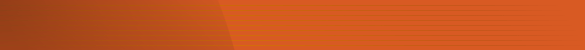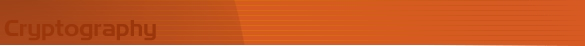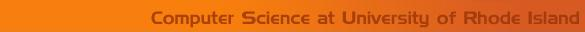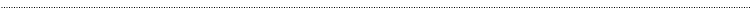Home Page Introduction Classical Ciphers Enigma Machine DES Key Agreement PGP R.S.A. Number Theory Other LinksPublickey CryptographyRSA

The RSA algorithm presented below was first published in 

Setup

1. Alice picks up two very large prime numbers, usually of order 100 decimal digits. These numbers are p and q.

2. Calculate, M and N, such that

1. M = p * q

2. N = (p-1) * (q-1)

3. Alice selects another number e, which is relatively prime to N.

4. Now she calculates d such that e * d = 1 (mod N).

5. Once p, q, M, N, e and d are selected, she publishes e and M. The rest of them are kept secret

1.  (e, M) is the public key

2. is the private key

Encryption and Decryption

Suppose Bob has a message to Alice. Regarding it, or a block of numbers in it, as a number x in the range of 0 to M-1.

Bob calculates the ciphertext as

y = xe mod M

Now Alice can decrypt it

x = yd mod M

Since Alice publishes e and M, any one who wants to send encrypted messages to Alice can do so, but these messages cannot be decrypted without the knowledge of d. d is kept secret and only Alice knows it, she can only decrypt messages.

Example

1. Choose p and q;  p =5 and q =11

2. Calculate M and N

M = p * q = 5 * 11 = 55

N = (p-1) * (q-1) = 4 * 10 = 40

1. Select e, e = 7

2. Calculate d

d = e-1 mod N = (1/7) mod 40 = 23

1. Let the message be x = 24

2. For encryption, y = xe mod M = (24 7 ) mod 55 = 4586471424 mod 55 = 29.

so the encrypted message is 29.

1. For decryption, x = yd mod M = (2923 ) mod 55 =

4316720717749415770740818372739989 mod 55 = 24

so the decrypted message is 24, which is the initial message